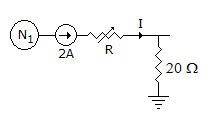# Electronics and Communication Engineering - Networks Analysis and Synthesis

1.

A series RLC circuit is supplied from a variable frequency ac source. The resonant frequency is ω0. The voltage across C is maximum at a frequency

 A. equal to ω0 B. slightly less than ω0 C. slightly more than ω0 D. none of the above

Explanation:

No answer description available for this question. Let us discuss.

2.

The maximum phase difference between the applied voltage and current in a RLC series current with finite values of R, L, C, and f can be

 A. less than 90° B. less than 45° C. 180° D. 30°

Explanation:

No answer description available for this question. Let us discuss.

3.

If figure, I = 2A if R = 20 Ω. When R = 10 Ω current I isA. 1 A B. 2 A C. 2.5 A D. 3 A

Explanation:

No answer description available for this question. Let us discuss.

4.

A phasor is

 A. a line representing the magnitude and direction of an alternating quantity B. a line which represents the magnitude and phase of an alternating quantity C. an instrument used for measuring phases of an unbalanced 3-phase load D. a coloured tag or band for distinguishing between different phases of a 3-phase supply

Explanation:

No answer description available for this question. Let us discuss.

5.

The phase difference (jω - p1 )

 A. phasor directed from p1 to jω B. phasor directed from origin to p1 C. phasor directed from origin to jω point D. phasor directed from jω to p1